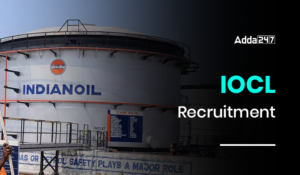Engineering Jobs   »   Quiz: Mechanical Engineering 11 May 2020

# Quiz: Mechanical Engineering 11 May 2020

Quiz: Mechanical Engineering
Exam: UPSSSC JE
Topic: Miscellaneous

Each question carries 1 mark
Negative marking: 1/4 mark
Time: 10 Minutes

Q1. The frictional resistance of a pipe varies approximately as
(a) Velocity of flow
(b) v^2
(c) √v
(d) v^(3/2)

Q2. Unit of viscosity is
(a) m^2/sec
(b) kg-sec/m^2
(c) N-sec/m^2
(d) N-sec^2/m

Q3. The bending moment on a section is maximum where shear force is
(a) Minimum
(b) Maximum
(c) Zero
(d) Changing sign

Q4. The condenser and evaporator tubes in a Freon refrigeration plant are made of
(a) Steel
(b) Copper
(c) Brass
(d) Aluminium

Q5. The ratio of ultimate stress and working stress is called
(a) Factor of safety
(b) Modulus of elasticity
(c) Poisson’s ratio
(d) None of the above

Q6. The value of frictional force is
(a) More than external force
(b) Equal to the external force
(c) Less than external force
(d) None of these

Q7. Efficiency of gas turbine can be increased by
(a) Reheating
(b) Inter-cooling
(d) All of the above

Q8. The volumetric efficiency of compressor with increase in compressor ratio will
(a) Increase
(b) Decrease
(c) Remain same
(d) None of the above

Q9. The law of parallelogram of forces gives the resultant of
(a) Parallel forces
(b) Two co-planar concurrent forces
(c) Like parallel forces
(d) Non co-planar concurrent forces

Q10. A particle acted upon by two forces in equilibrium, then the angle between the two forces is equal to
(a) 0°
(b) 45°
(c) 90°
(d) 180°

Solutions

S1. Ans.(b)
Sol. The frictional resistance of a pipe varies approximately as the square of the velocity of flow. It can be calculated as ∆P=(fLV^2)/(d.2g)

S2. Ans.(c)
Sol. We know that
Shear stress (τ)=μ(v/L)
Hence, unit of viscosity (μ) = N-sec/m^2

S3. Ans.(c)
Sol. We know that
dM/dX=F
For maximum bending moment (Mmax) at any section, dM/dX=0
So, Shear Force (F) = 0

S4. Ans.(b)
Sol. The condenser and evaporator tubes in a Freon refrigeration plant are made of copper alloy.

S5. Ans.(a)
Sol. Factor of safety is the ratio of ultimate stress to working stress.
Factor of safety=(Ultimate stress)/(Working stress)

S6. Ans.(b)
Sol. The value of frictional force is equal to the external force applied until it starts to move.
Maximum frictional force (F) =μN
Here, μ= static coefficient of friction
N= Normal force

S7. Ans.(c)
Sol. Regenerator is a device that is used to heat up compressed gases by exhaust gases (if the temperature of turbine outlet is higher than that of compressor outlet). By adding a regenerator in a gas turbine cycle, efficiency of gas turbine cycle increases due to increase in mean temperature of heat addition and decrease in mean temperature of heat rejection.

S8. Ans.(b)
Sol. The volumetric efficiency of a compressor is,
η=1+C-C(P2/P1)^(1/n)
By increasing compressor ratio, the volumetric efficiency will decrease.

S9. Ans.(b)
Sol. The law of parallelogram of forces gives the resultant of two co-planar concurrent forces.

S10. Ans.(d)
Sol. When the two forces acted on a particle and the particle is in equilibrium position, then the forces should be equal and opposite and hence, angle between the forces is 180°.

Sharing is caring!

•GATE Result 2023 Out, Download Result, C...
•GATE 2023 Admit Card Out, Download Link ...
•IOCL Recruitment 2023, Application Form,...
•DRDO Recruitment 2023, Notification, Vac...
•FCI JE Result 2023, Direct Link To Downl...
•NHAI Recruitment 2023 Out, Apply For 50 ...
•DFCCIL Cutoff 2023, Check DFCCIL Previou...
•How do I Get the Top Rank in GATE Exam?